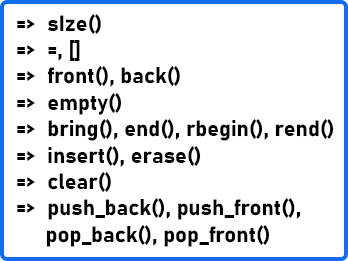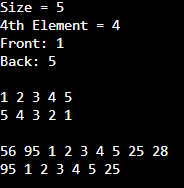# Deque in C++

## Deque in C++ with Examples:

In this article, I am going to discuss Deque Class Functions in C++ with Examples. Please read our previous article, where we discussed List Class Functions in C++ with Examples.

##### Deque in C++ with Examples:

Deque is another sequence container and it supports push and pop operations from both ends. This is not implemented to store elements continuously like a vector but here the implementation is slightly different. A typical implementation would be used i.e. fixed-size arrays. So, we have multiple fixed-size arrays. So, it is contiguous to some extent but we have many such arrays and these may not be contiguous. Here you can see the immediate advantage as compared to vectors.

In vectors, when this size is filled let us say this size was 10 at some point in time and we had copied 5 elements from the previous and 5 slots were remaining. So, once we insert 5 new elements, this will be full. And when we insert the 11th element, everything else needs to be copied to a new vector. So new space is allocated for 20. Those 10 elements have to be copied there and then the 11th one will be inserted there. Similarly, when this vector is full, again this copy will happen but in deque that is not the case.

In deque, once this is full, a new fixed-size array will be created and no more copies will happen. Just like other STL containers, you need to include a header file that is ‘deque’. It also supports random access so if you have a deque d and you want to find the third element or access the third element you can access that as d . The index starts from 0 and ends at the size – 1. So, all of these are much related. If you understand one container then you can use another one. You just need to know the limitations of that container and the operations that support it.

##### Deque Functions in C++:Let us understand each function in detail.

1. size() – it returns the size of the deque container associated with the function. If the container has no elements, then the function returns 0.
2. = – it is the assignment operator.
3. [] – This is the operator which provides you random access. You can provide the index in this operator; it will return the element at the given index.
4. front(), back() – The front gives you the first element and the back gives you the last element.
5. empty() – It returns a Boolean true or false depending on whether the container is empty or not.
6. begin(), end(), rbegin(), rend() – with these iterators you can traverse from left to right or right to left both ways. Begin is the iterator pointing to the first element and the end is the iterator pointing to one position or one slot past the last element. rbegins points to the last element and rend points one position before the first element. So, these are the iterators that help you to traverse in both directions.
7. Insert(), erase() – deque has insert and erases just like other containers. Insert provide a position or the iterator before which you want to insert. So, if we have an iterator that is pointing to let us say 5th element you want to insert some value then it will be inserted at the 5th position. So, whatever was the 5th element will be shifted to the 6th position. Similarly erase, you provide either the iterator from which you want to delete the element or you can also provide a range of values like from it1 to it2 excluding the last one.
8. Clear() – it will clear this entire container. So, everything will be cleared.
9. push_back() – it will append an element in the end. You have to pass a value here let’s say d.push_back (30) so it will add 40 in the end.
10. push_front() – new element will be pushed at the beginning i.e. v.push_front (50).
11. pop_back() – back will pop the last element
12. pop_front() – will pop the front element
##### Example to Understand Deque Class Functions in C++:
```#include <iostream>
#include <deque>

using namespace std;

void print_deque(deque<int> &deq){
for(deque<int>::iterator it = deq.begin(); it != deq.end(); ++it)
cout << *it << " ";
cout << endl;
}

void print_deque_reverse(deque<int> &deq){
for(deque<int>::reverse_iterator it = deq.rbegin(); it != deq.rend(); ++it)
cout << *it << " ";
cout << endl;
}

int main()
{
// create a deque
deque<int> d = {1, 2, 3, 4, 5};

cout << "Size = " << d.size() << endl; // print the size of deque deque
cout << "4th Element = " << d << endl; // print the 4th element of deque
cout << "Front: " << d.front() << endl; // print the first element of deque
cout << "Back: " << d.back() << endl; // print the last element of deque
cout << endl;

// print all the elements of deque
print_deque(d);

// print all the elements of deque in reverse order
print_deque_reverse(d);

cout << endl;

// adding elements from the last and front in the deque
d.push_back(25);
d.push_back(28);
d.push_front(95);
d.push_front(56);

print_deque(d);

// deleting element from the last and front in the deque
d.pop_back();
d.pop_front();

print_deque(d);

d.clear();

return 0;
}
```
###### Output: